﻿ A Shortened Recurrence Relation for Bernoulli NumbersPublications are Open
Access in this journal
Article Versions
Export Article
• Normal Style
• MLA Style
• APA Style
• Chicago Style
Research Article
Open Access Peer-reviewed

### A Shortened Recurrence Relation for Bernoulli Numbers

F. M. S. Lima
Turkish Journal of Analysis and Number Theory. 2018, 6(2), 49-51. DOI: 10.12691/tjant-6-2-3
Received January 13, 2018; Revised March 03, 2018; Accepted April 09, 2018

### Abstract

In this note, starting with a little-known result of Kuo, I derive a recurrence relation for the Bernoulli numbers B2n , n being a positive integer. This formula is shown to be advantageous in comparison to other known formulae for the exact symbolic computation of B2n. Interestingly, it is suitable for large values of n since it allows the computation of both B4n and B4n+2 from only B0, B2, ..., B2n.

### 1. Introduction

The Bernoulli numbers Bn, n being a nonnegative integer, can be defined by the generating function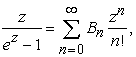(1)

which holds for all complex z with |z| < 2. The first few values are B0 = 1, B1 = -1/2, and B2 = 1/6. It is well-known that Bn = 0 for odd values of n, n>1. For even values of n, n>1, the numbers Bn form a subsequence of non-null rational numbers such that (-1)m+1 B2m>0 for all positive integer m. In other words, the entire subsequence of non-null Bernoulli numbers up to any Bn, n>1, consists of B0 and B1, given above, and the preceding numbers B2m, m = 1, ..., n/2. The basic properties of these numbers can be found, e.g., in Sec. 9.61 of 1.

The numbers Bn appear in many instances in pure and applied mathematics, most notably in number theory, finite differences calculations, and asymptotic analysis. They also appear as coefficients in the Euler-Maclaurin formula, thus being important for accurate numerical evaluation of special functions. Therefore, numerical computations of Bn are of great mathematical interest. To this end, recurrence formulae were soon recognized as the most efficient tool 2. One of the simplest such formulas is found by multiplying both sides of Eq. (1) by ex-1, using the Cauchy product with the Maclaurin series for ex-1, and equating the coefficients, which yields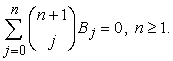(2)

This kind of recurrence has the disadvantage of demanding the previous knowledge of every B0, B1, ...,Bn-1 for the computation of Bn. On searching for more efficient formulae in literature, one finds shortened recurrence relations of two different types. The first type consists of lacunary recurrences, in which Bn is determined only from every second, or every third, etc., preceding Bn (see, e.g., the lacunary formula by Ramanujan in 3). The second type demands the knowledge of the second-half of Bernoulli numbers up to Bn-1 in order to compute Bn 4. For an extensive study of these and other recurrence relations involving the numbers Bn, see 5.

Here in this note, I apply the Euler's formula relating the even zeta value (2n) to B2n to a recurrence formula for (2n) obtained by Kuo in 6 in order to convert it into a new recurrence formula for B2n. This reveals a third type of recurrence for the Bernoulli numbers, in the sense that it allows us to compute both B4n and B4n+2 from only the first-half of the preceding numbers, i.e. B0, B2, ..., B2n.

### 2. Recurrence Relation for Bernoulli Numbers

For complex values of s with Re(s)>1, the Riemann zeta function is defined as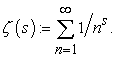In this domain, the convergence of this series is guaranteed by the integral test. For s=1, one has the well-known harmonic serie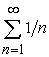, which diverges to infinity. For positive even values of s, one has a celebrated formula by Euler 7: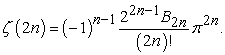(3)

Since (-1)n-1 B2n is a positive rational for all integer n>0, then (2n) is a positive rational multiple of 2n. The function (s), as defined above, can be extended to the entire complex plane (except for the only simple pole at s=1) by analytic continuation, which yields (0) = -1/2 8. Alternatively, we can use a globally convergent series representation due to Hasse (1930) 9: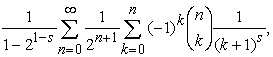(4)

valid for all complex s 1 +(2m)i/ln(2), m being an integer. At s=0, it reduces to (0) = -1/2, which shows that Eq. (3) is also valid for n=0.

These are the ingredients we need to state our first lemma, which comes from a little-known recurrence formula by Kuo (1949) 6, written here in terms of even zeta values.

Lemma 1 (Kuo's recurrence formula). For any positive integer n, one has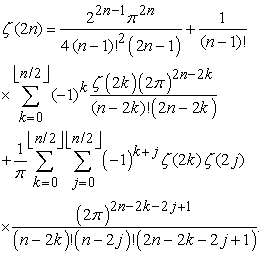This formula is proved in 6 by developing successive integrations (from 0 to x) of the Fourier series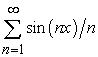, which converges to (-x)/2 for all x (0,2), and then applying Parseval's theorem.

We are now in a position to state and prove our main result. In what follows, (λ)n:= λ (λ+1) (λ+n-1) is the Pochhammer symbol.

Theorem 1 (Recurrence for B2n). For any integer n>0, the following formula holds: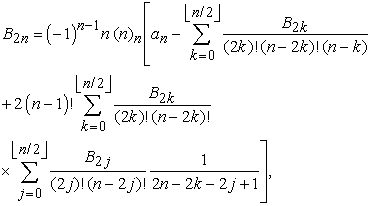where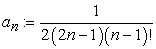Proof. On dividing both sides of Kuo's formula, our Lemma 1, by (2)2n, one finds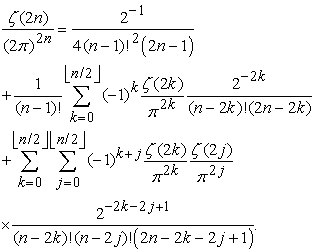(5)

From Eq. (3), we know that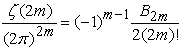, which is valid for all integer m 0. By substituting this in Eq. (5), one finds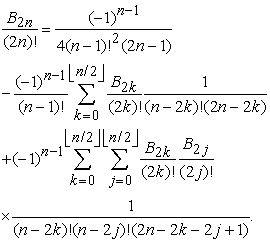(6)

Now, by factoring (-1)n-1 one finds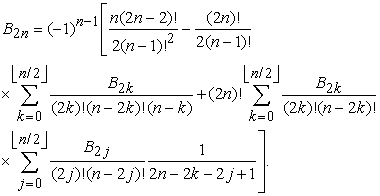(7)

On writing the first term in the right-hand side as (2n)!/[4(2n-1)(n-1)!2] and putting (2n)!/(n-1)! in evidence (out of the square brackets), the desired result follows.

For instance, when n=1, since (1)1 = 1 and B0=1, one readily finds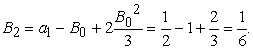(8)

For larger values of n, a more efficient, fast computation is achieved when one avoids the repeated computation of the factorials in our Theorem 1. For this, it is advantageous to make use of the algorithm given below. A short Maple code implementation of this algorithm is freely available in the journal website (file B4mB4mp2.mws).

Of course, more efficient numerical routines for floating-point computation of B2n can be found in some recent works (see, e.g., 10, 11, 12), but we are interested here only in exact symbolic computations of the rational numbers B2n, which are important for implementation in modern mathematical software such as Maple and Mathematica. Admittedly, most algorithms in 12 can, a priori, be implemented in a manner to yield exact symbolic results.

2.1. Algorithm

For completion, the algorithm corresponding to an efficient implementation of the recurrence formula given in our Theorem 1 is presented below.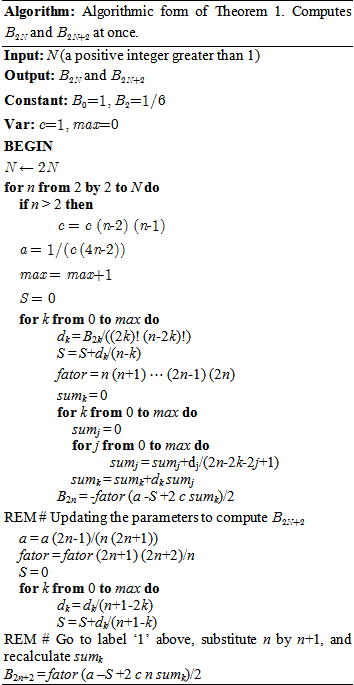### Acknowledgements

The author wishes to thank M. R. Javier for checking computationally all results in this work.

### References

  I.S. Gradshteyn and I.M. Rhyzik, Table of Integrals, Series, and Products, 7th ed., Academic Press, New York, 2007. In article View Article  J. Worpitzky, “Studien über die Bernoullischen und Eulerschen Zahlen,” J. Reine Angew. Math. 94, 203-232 (1883). In article View Article  S. Ramanujan, “Some Properties of Bernoulli's Numbers,” J. Indian Math. Soc. 3, 219-234 (1911). In article View Article  T. Agoh and K. Dilcher, “Shortened recurrence relations for Bernoulli numbers,” Discrete Math. 309, 887-898 (2009). In article View Article  K. Dilcher, L. Skula, I.Sh. Slavutskii, “Bernoulli numbers. Bibliography (1713-1990),” Queen's Papers in Pure and Applied Mathematics 87, Queen's University, Kingston, Ont., 1991. Updated on-line version: http://www.mathstat.dal.ca/~dilcher/bernoulli.html. In article View Article  H.-T. Kuo, “A recurrence formula for (2n),” Bull. Am. Math. Soc. 55, 573-574 (1949). In article View Article  L. Euler, “De summis serierum reciprocarum,” Commentarii Academiae Scientiarum Petropolitanae 7, 123-134 (1740). In article  E.C. Titchmarsh, The theory of the Riemann Zeta-function, 2nd ed., Clarendon Press, Oxford, 1986. In article View Article  H. Hasse, “Ein Summierungsverfahren für die Riemannsche Zeta-Reihe,” Math. Z. 32, 458-464 (1930). In article View Article  S. Akiyama and Y. Tanigawa, “Multiple Zeta Values at Non-Positive Integers,” Ramanujan J. 5, 327-351 (2001). In article View Article  R.P. Brent and P. Zimmermann, Modern computer arithmetic, Cambridge University Press, New York, 2010. Sec. 4.7.2. In article View Article  R.P. Brent and D. Harvey, “Fast computation of Bernoulli, Tangent and Secant numbers.” Springer Proceedings in Mathematics and Statistics, vol. 50, 127-142 (2013). In article View ArticleThis work is licensed under a Creative Commons Attribution 4.0 International License. To view a copy of this license, visit http://creativecommons.org/licenses/by/4.0/